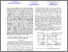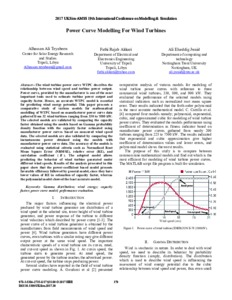# Power curve modelling for wind turbines

Teyabeen, A.A., Akkari, F.R. and , 2017. Power curve modelling for wind turbines. In: D. Al-Dabass, A. Orsoni, and G. Jenkins, eds., Proceedings of 2017 UKSim-AMSS 19th International Conference on Computer Modelling & Simulation, Cambridge, 5-7 April 2017. Piscataway, NJ: Institute of Electrical and Electronics Engineers, pp. 179-184. ISBN 9781538627358Preview
Text
15020_Jwaid.pdf - Published version

## Abstract

The wind turbine power curve WTPC describes the relationship between wind speed and turbine power output. Power curve, provided by the manufacturer is one of the most important tools used to estimate turbine power output and capacity factor. Hence, an accurate WTPC model is essential for predicting wind energy potential. This paper presents a comparative study of various models for mathematical modelling of WTPC based on manufacturer power curve data gathered from 32 wind turbines ranging from 330 to 7580 kW. The selected models are validated by comparing the capacity factor obtained using the models based on Gamma probability density function with the capacity factor estimated using manufacturer power curves based on measured wind speed data. The selected models are also validated by comparing the instantaneous power obtained using the models with manufacturer power curve data. The accuracy of the models is evaluated using statistical criteria such as Normalized Root Mean Square Error (NRMSE), relative error (RE), and correlation coefficient (R). The adopted model allows predicting the behavior of wind turbine generated under different wind speeds. Results of the analysis presented in this paper show that the power-coefficient based model presents favorable efficiency followed by general model, since they have lower values of RE in estimation of capacity factor, whereas the polynomial model showed the least accurate model.

Item Type: Chapter in book
Creators: Teyabeen, A.A., Akkari, F.R. and Jwaid, A.E.
Publisher: Institute of Electrical and Electronics Engineers
Place of Publication: Piscataway, NJ
Date: April 2017
ISBN: 9781538627358
Identifiers:
NumberType
10.1109/uksim.2017.30DOI
Divisions: Schools > School of Science and Technology
Record created by: Jonathan Gallacher
Date Added: 30 Sep 2019 14:26Edit View